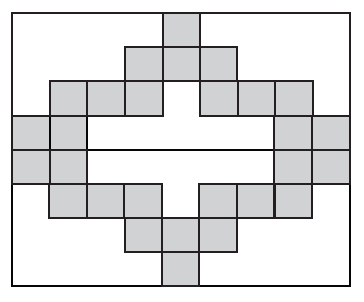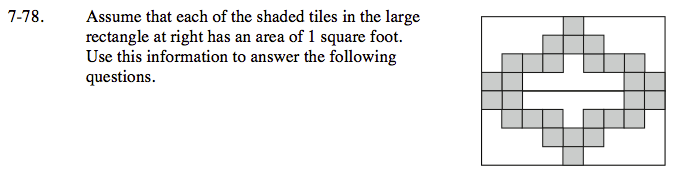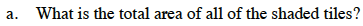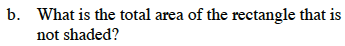### Home > CC1 > Chapter 7 > Lesson 7.2.4 > Problem7-78

7-78.
1.Assume that each of the shaded tiles in the large rectangle at right has an area of 1 square foot. Use this information to answer the following questions. Homework Help ✎

1. What is the total area of all of the shaded tiles?

2. What is the total area of the rectangle that is not shaded?If you know the number of shaded tiles and you know the area of each shaded tile, how can you find the total area of the shaded tiles?

1 square foot × 28 shaded tiles = 28 square feetUse the tiles to determine the length and width of the large rectangle.

Total unshaded area = total area − shaded area